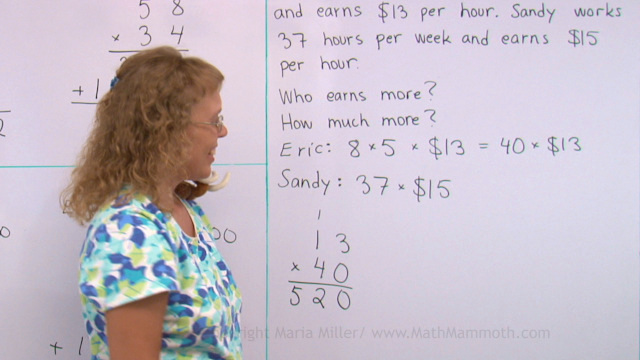^

# Videos for multi-digit multiplication (grades 4-5)

Find here free online math videos on multi-digit multiplication, meant for 4th and 5th grades.

These videos match the topics in Math Mammoth Multiplication 2 worktext (Blue Series)."Scales" (pan balance) problems (2 videos)

Multiply in parts 1 (partial products) – 2-digit by 1-digit multiplication

Multiply in parts 2 – partial products algorithm for 3-digit numbers and money amounts

Estimating in multiplication: round the factors!

Multiplying in columns, the standard way — 1-digit by 2,3,4-digit multiplication; standard algorithm

The order of operations again

So many of the same thing

Multiply by whole tens in columns — aka a shortcut for 2-digit multiplication when one factor is a multiple of ten

The standard multiplication algorithm with a two-digit multiplier — aka two-digit multiplication, and why we add a zero on the 2nd line (2 videos)

WAIT!

Receive my monthly collection of math tips & resources directly in your inbox — and get a FREE Math Mammoth book!You can unsubscribe at any time.

### Math Mammoth Tour

Confused about the different options? Take a virtual email tour around Math Mammoth! You'll receive:

An initial email to download your GIFT of over 400 free worksheets and sample pages from my books. Six other "TOURSTOP" emails that explain the important things and commonly asked questions concerning Math Mammoth curriculum. (Find out the differences between all these different-colored series!)

This way, you'll have time to digest the information over one or two weeks, plus an opportunity to ask me personally about the curriculum.
A monthly collection of math teaching tips & Math Mammoth updates (unsubscribe any time)### "Mini" Math Teaching Course

This is a little "virtual" 2-week course, where you will receive emails on important topics on teaching math, including:

- How to help a student who is behind
- Troubles with word problems
- Teaching multiplication tables
- Why fractions are so difficult
- The value of mistakes
- Should you use timed tests
- And more!

A GIFT of over 400 free worksheets and sample pages from my books right in the very beginning.A monthly collection of math teaching tips & Math Mammoth updates (unsubscribe any time)
Enter your email to receive math teaching tips, resources, Math Mammoth news & sales, humor, and more! I tend to send out these tips about once monthly, near the beginning of the month, but occasionally you may hear from me twice per month (and sometimes less often).• A GIFT of over 400 free worksheets and sample pages from my books.